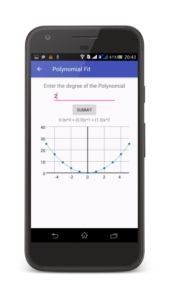# Polynomial Fitting – JAVA Code/Program (works on Android as well)

Okay, so the following is a code for fitting a polynomial to a given set of data using the Least Squares Approximation Method(Wikipedia).

Formula Used:$\begin{bmatrix} N& \Sigma x_i& \Sigma x_i^2& \dots& \Sigma x_i^n& \\ \Sigma x_i& \Sigma x_i^2& \Sigma x_i^3& \dots& \Sigma x_i^{n+1}& \\ \Sigma x_i^2& \Sigma x_i^3& \Sigma x_i^4& \dots& \Sigma x_i^{n+2}& \\ & \vdots& & & \vdots& \\ \Sigma x_i^n& \Sigma x_i^{n+1}& \Sigma x_i^{n+2}& \dots& \Sigma x_i^{2n}& \end{bmatrix}\begin{bmatrix} a \end{bmatrix}=\begin{bmatrix} \Sigma y_i\\ \Sigma x_iy_i\\ \Sigma x_i^2y_i\\ \vdots\\ \Sigma x_i^ny_i \end{bmatrix}$
where$x_i$ and$y_i$ are the data-points entered by the user.

I had written a C++ code for this a long time ago, and coincidentally it got very popular for some reason. But then I felt the need to make an Android app that does the same.

So I ported my code to JAVA so that it works in my Android App.

Let’s say you have x-axis values and y-axis values in two double arrays called x[] and y[] of size N(no. of data points). You can either have the user enter them or have them imported from a CSV. It’s your call.
Then you need to have the user enter the degree(order) of the polynomial to use to fit the curve.
Note: If you have n points then an n-degree polynomial will interpolate(fit your data perfectly) your data.

The following information is required:

int n;                       //degree of polynomial to fit the data
int N;                       //no. of data points
double[] x=double[];         //array to store x-axis data points
double[] y=double[];         //array to store y-axis data points


Once you have all the values for x[], y[], n, and N, the following code will fit a polynomial of nth degree to the given set of data-points and return the coefficients of the polynomial in an array a[], such that a is the coefficient of x^0 , a is the coefficient of x^1,… and so on.


double X[] = new double[2 * n + 1];
for (int i = 0; i < 2 * n + 1; i++) {
X[i] = 0;
for (int j = 0; j < N; j++)
X[i] = X[i] + Math.pow(x[j], i);        //consecutive positions of the array will store N,sigma(xi),sigma(xi^2),sigma(xi^3)....sigma(xi^2n)
}
double B[][] = new double[n + 1][n + 2], a[] = new double[n + 1];            //B is the Normal matrix(augmented) that will store the equations, 'a' is for value of the final coefficients
for (int i = 0; i <= n; i++)
for (int j = 0; j <= n; j++)
B[i][j] = X[i + j];            //Build the Normal matrix by storing the corresponding coefficients at the right positions except the last column of the matrix
double Y[] = new double[n + 1];                    //Array to store the values of sigma(yi),sigma(xi*yi),sigma(xi^2*yi)...sigma(xi^n*yi)
for (int i = 0; i < n + 1; i++) {
Y[i] = 0;
for (int j = 0; j < N; j++)
Y[i] = Y[i] + Math.pow(x[j], i) * y[j];        //consecutive positions will store sigma(yi),sigma(xi*yi),sigma(xi^2*yi)...sigma(xi^n*yi)
}
for (int i = 0; i <= n; i++)
B[i][n + 1] = Y[i];                //load the values of Y as the last column of B(Normal Matrix but augmented)
n = n + 1;
for (int i = 0; i < n; i++)                    //From now Gaussian Elimination starts(can be ignored) to solve the set of linear equations (Pivotisation)
for (int k = i + 1; k < n; k++)
if (B[i][i] < B[k][i])
for (int j = 0; j <= n; j++) {
double temp = B[i][j];
B[i][j] = B[k][j];
B[k][j] = temp;
}

for (int i = 0; i < n - 1; i++)            //loop to perform the gauss elimination
for (int k = i + 1; k < n; k++) {
double t = B[k][i] / B[i][i];
for (int j = 0; j <= n; j++)
B[k][j] = B[k][j] - t * B[i][j];    //make the elements below the pivot elements equal to zero or elimnate the variables
}
for (int i = n - 1; i >= 0; i--)                //back-substitution
{                        //x is an array whose values correspond to the values of x,y,z..
a[i] = B[i][n];                //make the variable to be calculated equal to the rhs of the last equation
for (int j = 0; j < n; j++)
if (j != i)            //then subtract all the lhs values except the coefficient of the variable whose value                                   is being calculated
a[i] = a[i] - B[i][j] * a[j];
a[i] = a[i] / B[i][i];            //now finally divide the rhs by the coefficient of the variable to be calculated
}


Well, that’s it. The array a[] contains the coefficients such that a[i]=coefficient of x^i.

To understand the theory behind this, refer to this link.Hope you guys find it useful!
If you have any questions/doubts, hit me up in the comments section below.

You can refer to the following links for more info:
Linear Fitting – Lab Write-Up
Linear Fitting – C++ Program
Linear Fitting – Scilab Code
Curve Fit Tools – Android App (using the above code)
Curve Fit Tools – Documentation
Curve Fit Tools – Play Store
Curve Fit Tools – GitHub Repository
Curve Fitters – Scilab Toolbox[wpedon id="7041" align="center"]

## One thought on “Polynomial Fitting – JAVA Code/Program (works on Android as well)”

1. Thanks. You saved me a lot of time.